## Vector Definition Using Initial and Final Values...

A simple question:

Can I define a Vector using:

a) Initial Value (a), Final Value (b) and the number of elements (N) (assuming linear spacing)
For example: Starts at 1, finishes at 12 with 5 elements:

[ 1 , 3.75 , 6.5 , 9.25 , 12 ]

b) Initial Value (a), Final Value (b) and step (s)
For example: Starts at 2, finishes at 6 with a step of 0.5

[ 2 , 2.5 , 3 , 3.5 , 4 , 4.5 , 5 , 5.5 , 6]

but using only a,b and N ; or a,b and s directly?

Thanks.

------
------

I know this can be done using the seq command and some basic definitions:

In the first case:
Vector([ seq(a+(b-a)/N*i,i=0..N) ]); should do the trick

And the second case is even easier:
Vector([seq(i,i=2..6,0.5)]);

PS. I know for the second case that using any arbitrary step can result in loosing the final value "b" of the sequence because you won't step on b using that arbitrary step value.

As I said above. I just want to know if there is a direct way to define these cases.

## Does maple have a hash map type?...

Does Maple have a built in hash map (associative array) datatype. I need to write a program where I will be iteratively building a lookup table. It will be very convenient for me if Maple has a hash map map structure built in.

## Is it possible to capture theta, phi and psi ?...

Hi,

Is it possible to capture programatically the values of theta, phi and psi when you manually rotate a 3D plot?

PS: The target version is Maple 2020

## how to simplify sqrt(u)=1/sqrt(u)?...

sometimes I get equations where there is clearly more simplification on it. For an example

```restart;
ode:=y(x)=diff(y(x),x)^3*y(x)^2+2*x*diff(y(x),x);
new_ode:=PDEtools:-dchange({y(x)=sqrt(u(x))},ode,{u});
```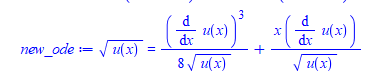Doing this by hand, I would now write the above as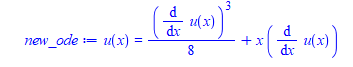by multiplying both sides by sqrt(u(x))

```new_ode :=sqrt(u(x))*lhs(new_ode)=simplify(sqrt(u(x))*rhs(new_ode))
```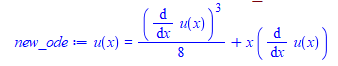Since I am doing this in a program, without knowing what the expression is, I need to have this simplification done automatically, since I do not know before hand, if the resulting expression has this form or not. So I automatically call simplify on it.

I tried on a simpler example

`expr:=sqrt(u)=1/sqrt(u)`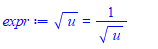And want to simplify this to u=1

I can't do

```new_expr :=sqrt(u)*lhs(expr)=sqrt(u)*rhs(expr)
```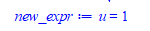Since this is done in a program, without the benefit of looking first and then deciding what to do. Everything needs to be automated since the expression can be anything.

So I need a generic operation to apply and have Maple simplify it, if this pattern exist.  I tried many things, but do far nothing is working:

```restart;
expr:=sqrt(u)=1/sqrt(u);
expand(expr);
expand(expr) assuming real;
combine(expr);
combine(expr,sqrt);
combine(expr,power);
expand(expr);
collect(expr,sqrt(u));
collect(lhs(expr)-rhs(expr)=0,sqrt(u));
simplify(lhs(expr)-rhs(expr)=0) assuming u<>0; # I expected this to work
simplify(expr) assuming real;
simplify(expr,size=false);
simplify(expr,sqrt) assuming real;
simplify(expr,sqrt) assuming positive;
simplify(expr,sqrt,symbolic) assuming positive;
simplify(expr,sqrt,symbolic,size=false) assuming positive;
simplify(expr,symbolic,size=false);
simplify(expr) assuming u<>0;
rationalize(expr);

```

no change.

Please do not give me an answer that requires one to use specific solution assuming one can see the expression. The solution needs to be something generic and work on any such expression that I can apply to any expression and have Maple simplify the sqrts on both sides, if they are there, since this is done in a program.

From the above, this should have worked

```
expr:=sqrt(u)=1/sqrt(u);
simplify(lhs(expr)-rhs(expr)=0) assuming u<>0;
simplify(lhs(expr)-rhs(expr)=0,symbolic);
```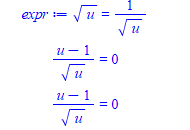Using Mathematica

```ClearAll[u];
expr = Sqrt[u] == 1/Sqrt[u]
Simplify[expr[] - expr[] == 0, Assumptions -> u != 0]
```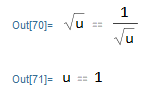Any suggestions? I am sure there is a simple way to do this in Maple, I just have not found it yet.

Maple 2020.2

## how to simplify this expression to zero?...

Why Maple can't simplify this expression to zero?

```restart;
ode:=diff(y(x),x)-y(x) = x*y(x)^(1/2);
ic:=y(0)=4;
sol:=dsolve([ode, ic],y(x));
check:=odetest(sol,ode);
```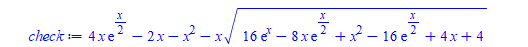`simplify(check) assuming x>0`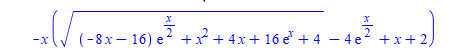In Mathematica:

```ClearAll[x]
check = -2*x + 4*x*Exp[x/2] - x^2 -x*Sqrt[x^2 - 8*x*Exp[x/2] + 4*x + 16*Exp[x] - 16*Exp[x/2] + 4]
Simplify[check, Assumptions -> x > 0]```